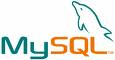User-defined functions in sql for mysql pay a huge performance penalty. Say you define a simple function like the following:

delimiter // create function delta (n bigint, uniq int) returns float deterministic begin declare last_n bigint; return n; end // delimiter ;

Guess how long it takes to run that query over a 100K row table? Let's compare it to a simple sum().

mysql> select sum(foo) from benchtest; +----------+ | sum(foo) | +----------+ | 100000 | +----------+ 1 row in set (0.10 sec) mysql> select sum(delta(foo,1)) from benchtest; +-------------------+ | sum(delta(foo,1)) | +-------------------+ | 0 | +-------------------+ 1 row in set (1.41 sec)

It pays to keep functions to a bare minimum. I've split delta() into two functions: delta_init() and delta(). And with that, delta() has the following run-time on a 100K table:

mysql> select sum(delta(foo,1)) from benchtest; +-------------------+ | sum(delta(foo,1)) | +-------------------+ | 0 | +-------------------+ 1 row in set (7.30 sec)

Which just makes me wince; while fine for small selects (currently running on ~500 rows), this definitely needs some more love. I'll try converting it to a c-based UDF next.

delimiter // create function delta_init () returns integer begin create temporary table if not exists tmp_delta ( id int primary key, last bigint ); return 0; end // delimiter ; drop function delta; delimiter // create function delta (n bigint, uniq int) returns float deterministic begin declare last_n bigint; -- first datum always returns 0 or garbage set last_n = n; select last into last_n from tmp_delta where id = uniq ; replace into tmp_delta set last = n , id = uniq; return n - last_n; end // delimiter ;